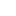### Field in the vicinity of the axis of a uniformly charged hoop - Corrected Exercises Gauss Theorem

A hoop of radius R, center O, carries the linear  uniform load λ.
1.         Determining the expression of the electrostatic field created by the hoop at a point M of the axis Oz.
2.         It is proposed to calculate now the field in the vicinity of the axis of the hoop.
Using a Gaussian surface having the form of a small Oz axis of cylinder of radius r and of length dz and by assuming that E z (r, z)= E z (axis) to a point close to the axis, show that the radial component of the field is related to the value of the field axis by:

Determine